# NCERT Solutions for Class 8 Maths Chapter 11 Exercise 11.3 – Mensuration

NCERT Solutions for Class 8 Maths Chapter 11 Exercise 11.3 – Mensuration, has been designed by the NCERT to test the knowledge of the student on the following topics:-

• Solid Shapes
• Surface Area of Cube, Cuboid and Cylinder
– Cuboid
– Cube
– Cylinders

### NCERT Solutions for Class 8 Maths Chapter 11 Exercise 11.3 – Mensuration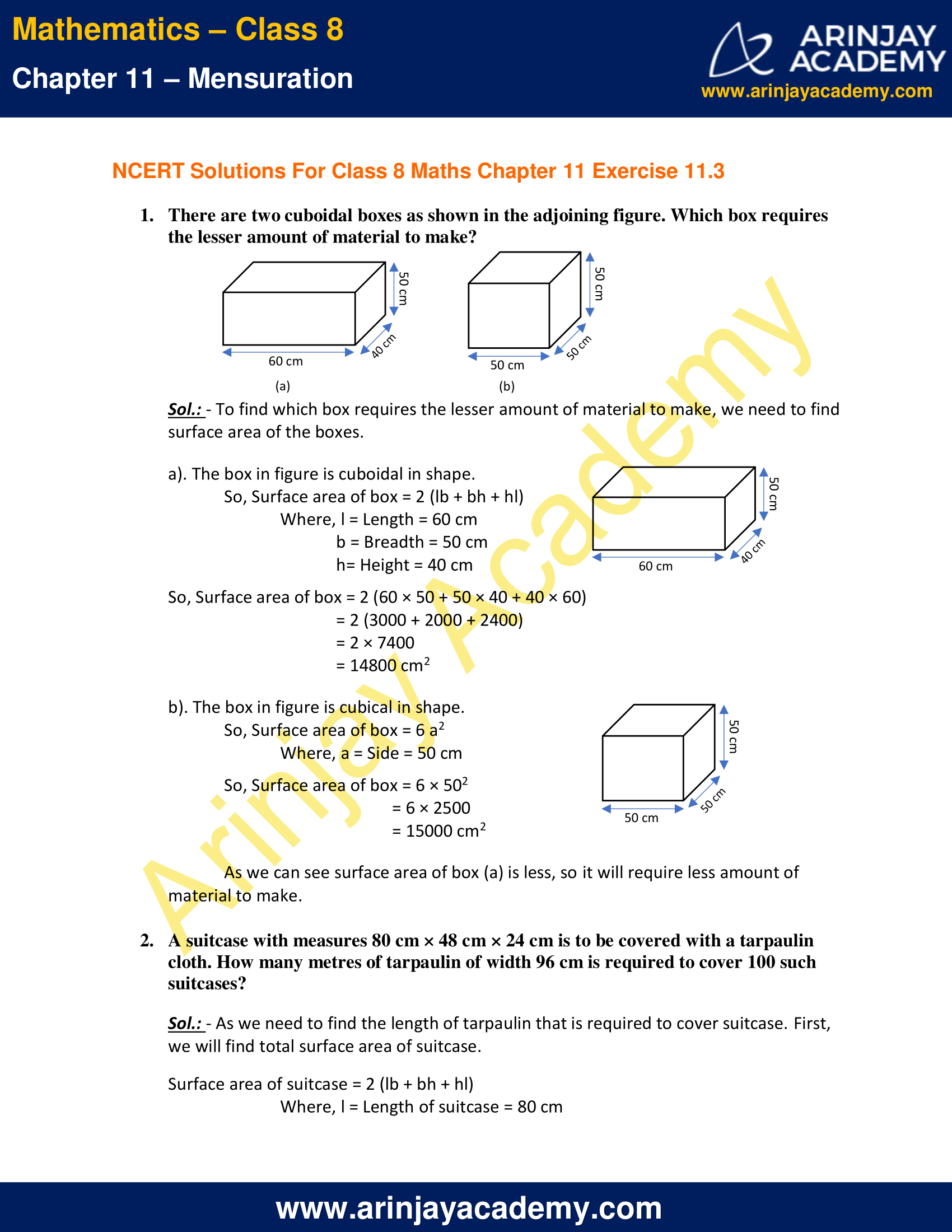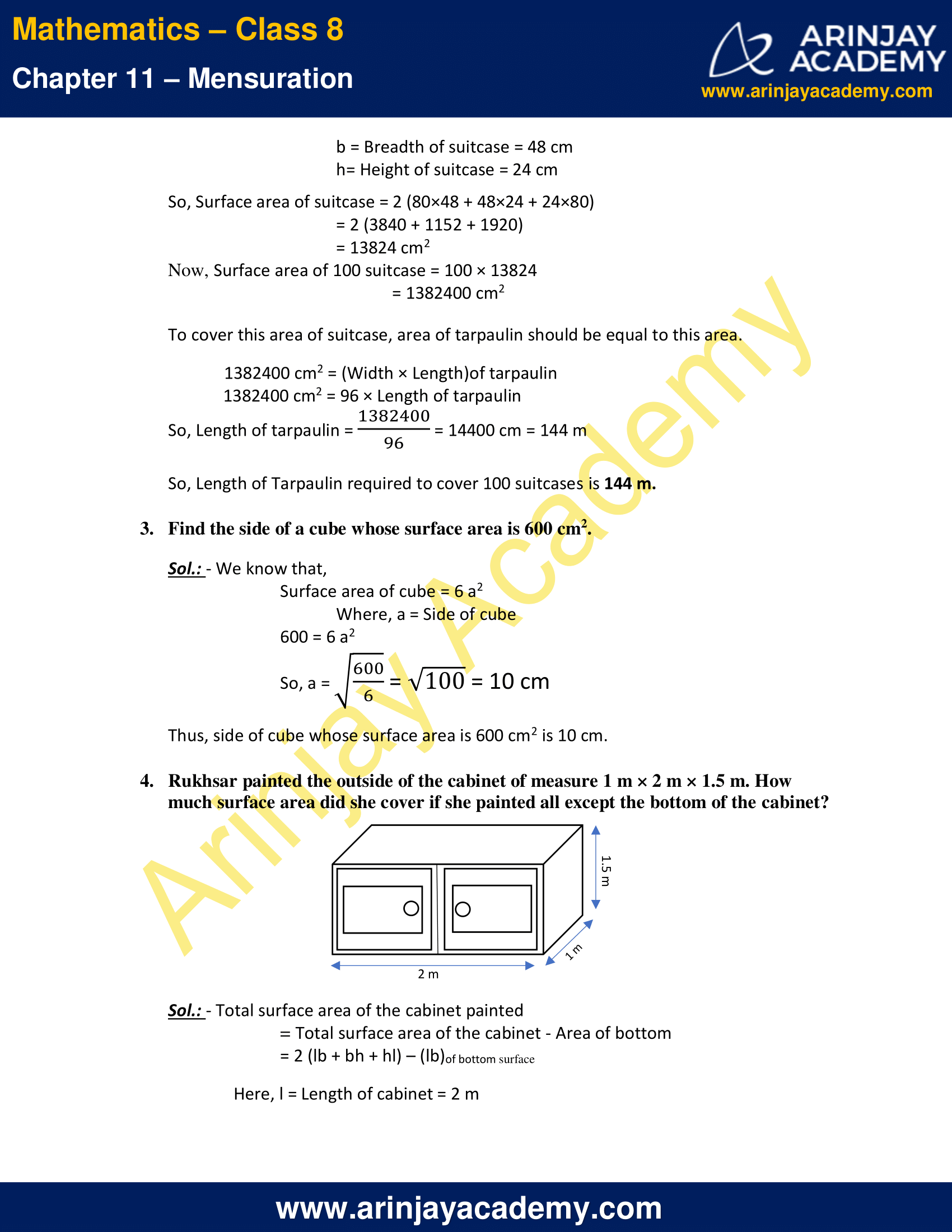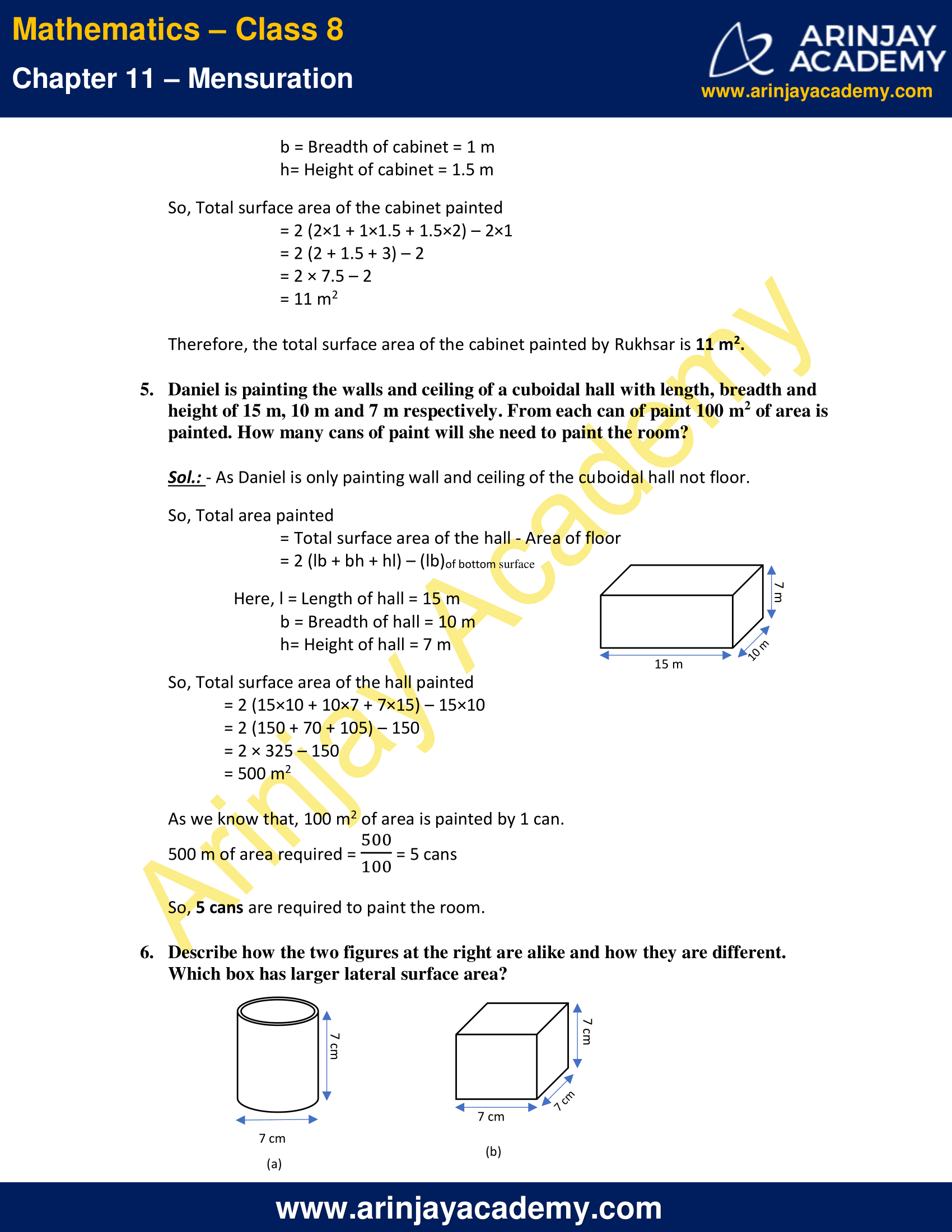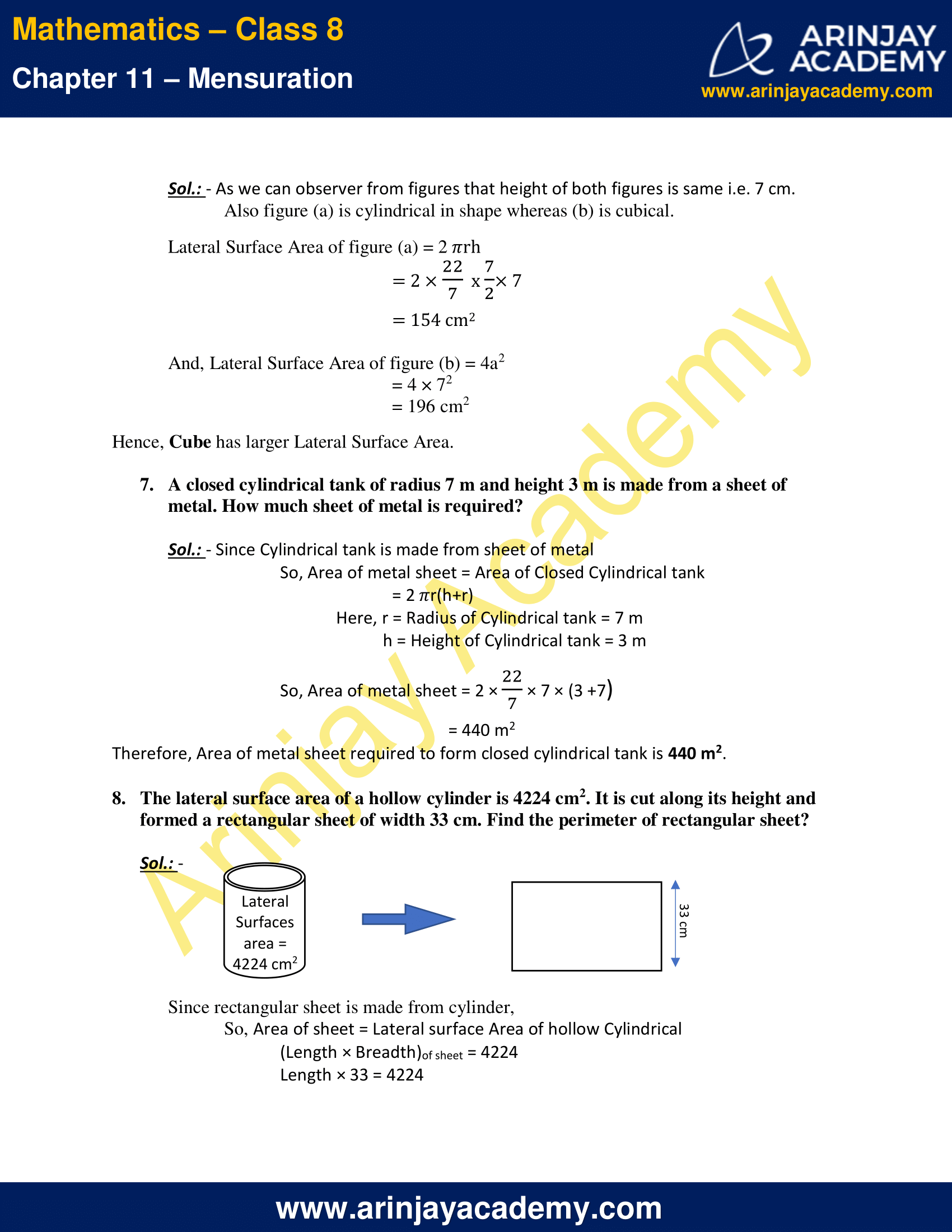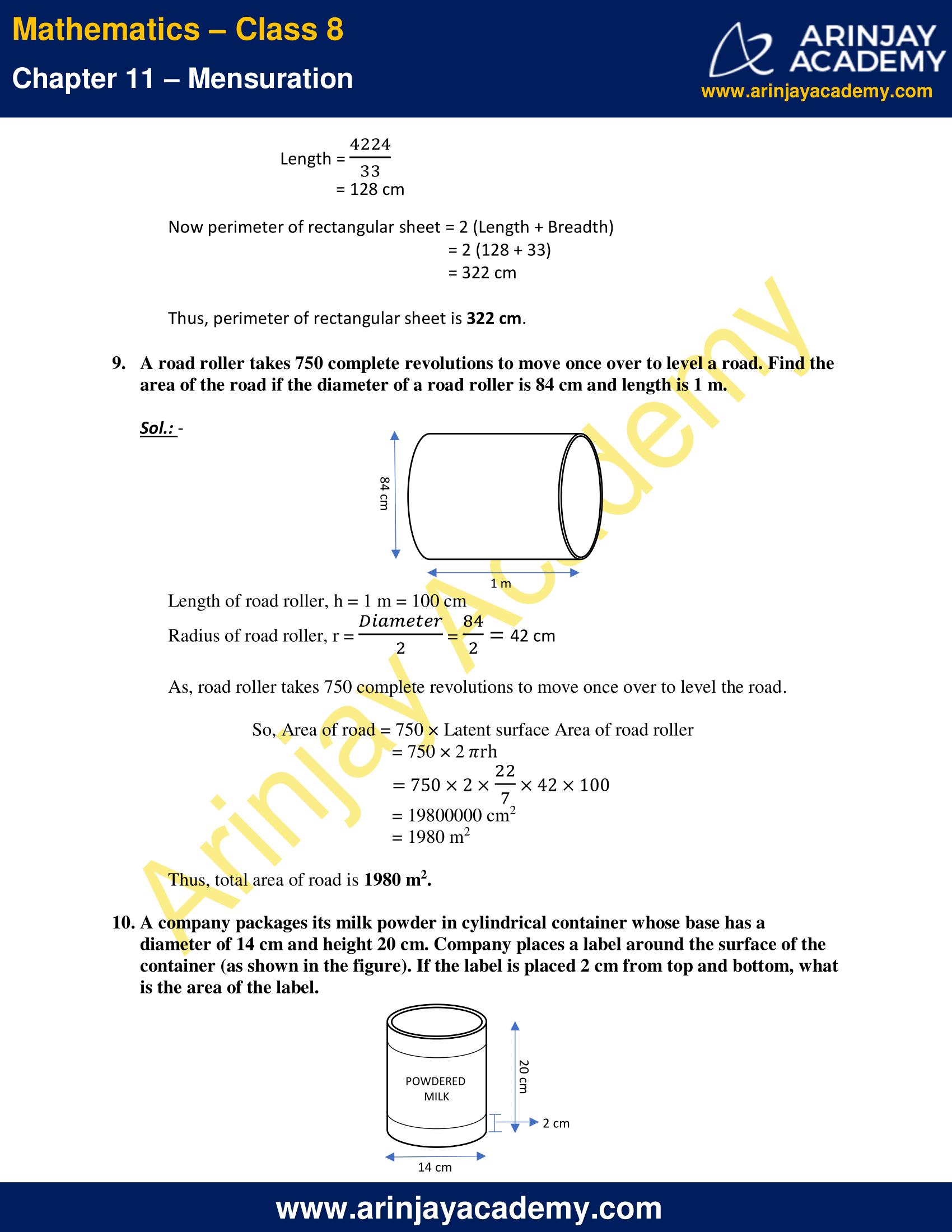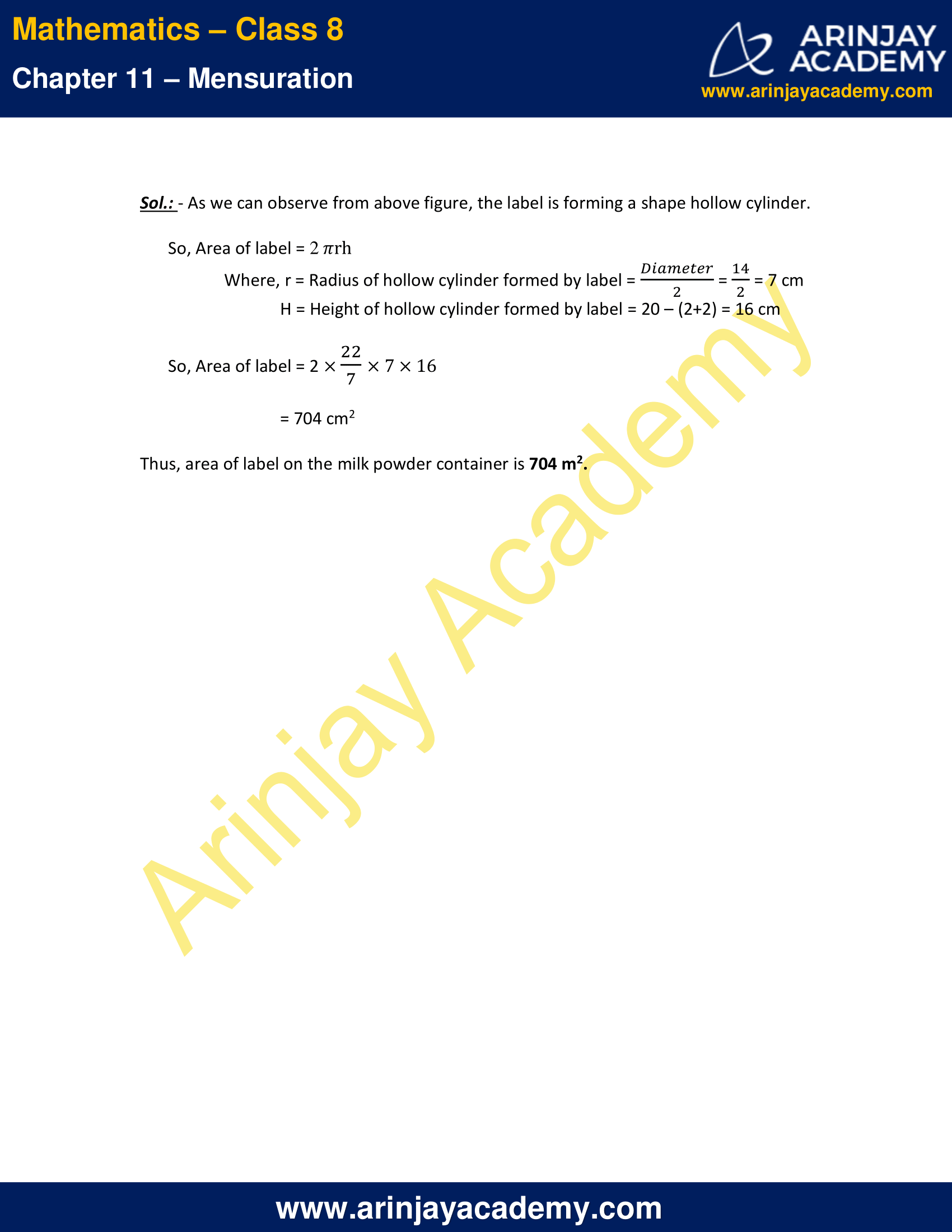NCERT Solutions for Class 8 Maths Chapter 11 Exercise 11.3 – Mensuration

1. There are two cuboidal boxes as shown in the adjoining figure. Which box requires the lesser amount of material to make?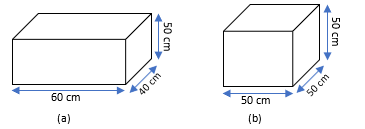Sol.: – To find which box requires the lesser amount of material to make, we need to find surface area of the boxes.

a). The box in figure is cuboidal in shape.
So, Surface area of box = 2 (lb + bh + hl)
Where, l = Length = 60 cm
b = Breadth = 50 cm
h= Height = 40 cm

So, Surface area of box = 2 (60 × 50 + 50 × 40 + 40 × 60)
= 2 (3000 + 2000 + 2400)
= 2 × 7400
= 14800 cm2

b). The box in figure is cubical in shape.
So, Surface area of box = 6 a2
Where, a = Side = 50 cm
So, Surface area of box = 6 × 502
= 6 × 2500
= 15000 cm2

As we can see surface area of box (a) is less, so it will require less amount of material to make.

2. A suitcase with measures 80 cm × 48 cm × 24 cm is to be covered with a tarpaulin cloth. How many metres of tarpaulin of width 96 cm is required to cover 100 such suitcases?

Sol.: – As we need to find the length of tarpaulin that is required to cover suitcase. First, we will find total surface area of suitcase.
Surface area of suitcase = 2 (lb + bh + hl)
Where, l = Length of suitcase = 80 cm
b = Breadth of suitcase = 48 cm
h= Height of suitcase = 24 cm

So, Surface area of suitcase = 2 (80×48 + 48×24 + 24×80)
= 2 (3840 + 1152 + 1920)
= 13824 cm2
Now, Surface area of 100 suitcase = 100 × 13824
= 1382400 cm2

To cover this area of suitcase, area of tarpaulin should be equal to this area.
1382400 cm2 = (Width × Length)of tarpaulin
1382400 cm2 = 96 × Length of tarpaulin
So, Length of tarpaulin = (1382400/96) = 14400 cm = 144 m
So, Length of Tarpaulin required to cover 100 suitcases is 144 m.

3. Find the side of a cube whose surface area is 600 cm2.

Sol.: – We know that,
Surface area of cube = 6 a2
Where, a = Side of cube
600 = 6 a2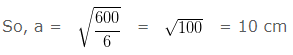Thus, side of cube whose surface area is 600 cm2 is 10 cm.

4. Rukhsar painted the outside of the cabinet of measure 1 m × 2 m × 1.5 m. How much surface area did she cover if she painted all except the bottom of the cabinet?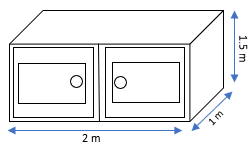Sol.: – Total surface area of the cabinet painted = Total surface area of the cabinet – Area of bottom
= 2 (lb + bh + hl) – (lb)of bottom surface
Here, l = Length of cabinet = 2 m
b = Breadth of cabinet = 1 m
h= Height of cabinet = 1.5 m

So, Total surface area of the cabinet painted
= 2 (2×1 + 1×1.5 + 1.5×2) – 2×1
= 2 (2 + 1.5 + 3) – 2
= 2 × 7.5 – 2
= 11 m2

Therefore, the total surface area of the cabinet painted by Rukhsar is 11 m2.

5. Daniel is painting the walls and ceiling of a cuboidal hall with length, breadth and height of 15 m, 10 m and 7 m respectively. From each can of paint 100 m2 of area is painted. How many cans of paint will she need to paint the room?

Sol.: – As Daniel is only painting wall and ceiling of the cuboidal hall not floor.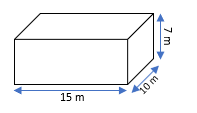So, Total area painted
= Total surface area of the hall – Area of floor
= 2 (lb + bh + hl) – (lb)of bottom surface

Here, l = Length of hall = 15 m
b = Breadth of hall = 10 m
h= Height of hall = 7 m

So, Total surface area of the hall painted
= 2 (15×10 + 10×7 + 7×15) – 15×10
= 2 (150 + 70 + 105) – 150
= 2 × 325 – 150
= 500 m2

As we know that, 100 m2 of area is painted by 1 can.
500 m of area required = (500/100) = 5 cans

So, 5 cans are required to paint the room.

6. Describe how the two figures at the right are alike and how they are different. Which box has larger lateral surface area?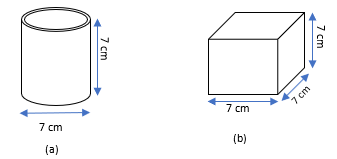Sol.: – As we can observer from figures that height of both figures is same i.e. 7 cm.
Also figure (a) is cylindrical in shape whereas (b) is cubical.

Lateral Surface Area of figure (a) = 2 ?rh
= 2 × 22/7  x 7/2 × 7
= 154 cm2

And, Lateral Surface Area of figure (b) = 4a2
= 4 × 72
= 196 cm2

Hence, Cube has larger Lateral Surface Area.

7. A closed cylindrical tank of radius 7 m and height 3 m is made from a sheet of metal. How much sheet of metal is required?

Sol.: – Since Cylindrical tank is made from sheet of metal
So, Area of metal sheet = Area of Closed Cylindrical tank
= 2 ?r(h+r)
Here, r = Radius of Cylindrical tank = 7 m
h = Height of Cylindrical tank = 3 m
So, Area of metal sheet = 2 × (22/7) × 7 × (3 +7)
= 440 m2
Therefore, Area of metal sheet required to form closed cylindrical tank is 440 m2.

8. The lateral surface area of a hollow cylinder is 4224 cm2. It is cut along its height and formed a rectangular sheet of width 33 cm. Find the perimeter of rectangular sheet?

Sol.: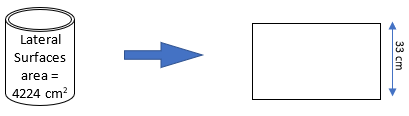Since rectangular sheet is made from cylinder,
So, Area of sheet = Lateral surface Area of hollow Cylindrical
(Length × Breadth)of sheet = 4224
Length × 33 = 4224
Length = (4224/33)
= 128 cm

Now perimeter of rectangular sheet = 2 (Length + Breadth)
= 2 (128 + 33)
= 322 cm

Thus, perimeter of rectangular sheet is 322 cm.

9. A road roller takes 750 complete revolutions to move once over to level a road. Find the area of the road if the diameter of a road roller is 84 cm and length is 1 m.

Sol.: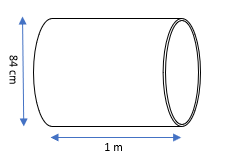Length of road roller, h = 1 m = 100 cm
Radius of road roller, r = Diameter/2 = 84/2 = 42 cm

As, road roller takes 750 complete revolutions to move once over to level the road.

So, Area of road = 750 × Latent surface Area of road roller
= 750 × 2 ?rh
= 750 × 2 × (22/7) × 42 × 100
= 19800000 cm2
= 1980 m2

Thus, total area of road is 1980 m2.

10. A company packages its milk powder in cylindrical container whose base has a diameter of 14 cm and height 20 cm. Company places a label around the surface of the container (as shown in the figure). If the label is placed 2 cm from top and bottom, what is the area of the label.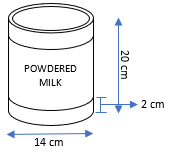Sol.: – As we can observe from above figure, the label is forming a shape hollow cylinder.
So, Area of label = 2 ?rh
Where, r = Radius of hollow cylinder formed by label = Diameter/2 = 14/2 = 7 cm
H = Height of hollow cylinder formed by label = 20 – (2+2) = 16 cm

So, Area of label = 2 × (22/7) × 7 × 16
= 704 cm2

Thus, area of label on the milk powder container is 704 m2.

The next Exercise for NCERT Solutions for Class 8 Maths Chapter 11 Exercise 11.4 – Mensuration can be accessed by clicking here

Download NCERT Solutions for Class 8 Maths Chapter 11 Exercise 11.3 – Mensuration